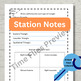# TrianglesSubject
Resource Type
File Type

PDF

(1 MB|41 pages)
Also included in:
1. This is a unit introducing the important aspects of triangles. This unit includes lessons covering concurrent segments, congruent triangles, types of triangles and the Pythagorean Theorem. All lessons come with notes, practice and assessments. The concurrent segment resource contains a project. You
\$21.25
\$17.00
Save \$4.25
2. This is a complete High School Geometry Curriculum. You will receive a combination of worksheets, notes, quizzes, tests, projects and digital activities. I am a Texas teacher and I have tried my best to include as many objectives from other states in this curriculum. If you find something is missing
\$189.70
\$132.79
Save \$56.91
Product Description

This Triangle unit is a catch all! You can fulfill many goals with this lesson unit. Here's what your get:

***Review of Triangles from previous grades,

***Triangle Sum Proof which is a Common Core and TEK objective

***Constructions and explorations with patty paper

***hands-on activity

***stations for collaboration and differentiation

***lesson plan

***assignments for practice

***assessment

What geometry is covered you ask?

***Names of triangles

***Triangle Sum Theorem

***Exterior Angle Theorem

***Using algebra to find missing sides and angles

***What side lengths work for triangles

***Isosceles Triangle basics - parts of the triangle

***Equilateral Triangle basics

***Naming Triangles with respect to sides and angles and drawing an isosceles right etc.

Enjoy this resource and don't forget to follow me and check out all my products!

This resource is also in my Geometry Curriculum

Other items in the Geometry Curriculum:

1. Intro to Geometry: Undefined Terms

3. Distance and Midpoint Activity with Zombie Problem

4. Inductive Vs. Deductive

5. Conditional Statements

6. Geometry Proof

7. Geometry Exam 1 and 8. Exam 1 Review

9. Parallel Lines and Transversals {With Project}

10. Parallel Lines and Perpendicular Lines Activities and Quiz

11. Geometry: Transformations

12. Congruent Triangle Exploration

13. Concurrent Segments in Triangles {With a Project}

14. Geometry Exam 2

15. Intro to Triangles

16. Pythagorean Theorem and Its Converse

18. Fall Semester Final {Editable}

2nd Semester Starts Here:

19. Similar Triangle Unit}

20. Right Triangles and Geometric Mean

21. Special Right Triangles

22. Right Triangle Test

23. Circles - Special Angles and Segment Properties

Exponential Function Bundle:

Exponential Functions Bundle

Are You College Ready Packet 1: Pemdas, Fractions, Expressions and More

Are You College Ready Packet 2: Equations, Inequalities, Graphing and More

Are You College Ready Packet 6: Transformations, Isosceles Triangles and More

Are You College Ready Packet 7: Probability and Statistics

ACT - Math Test Prep is here:

ACT Math Test Prep Bundle

ACT Math Test Prep 1

ACT Math Test Prep 2

ACT Math Test Prep 3

Data Driven Classroom Instruction: Reteaching Weak Objectives

Circles: Area, Circumference Coloring Activity

Total Pages
41 pages
Included
Teaching Duration
3 days
Report this Resource to TpT
Reported resources will be reviewed by our team. Report this resource to let us know if this resource violates TpT’s content guidelines.